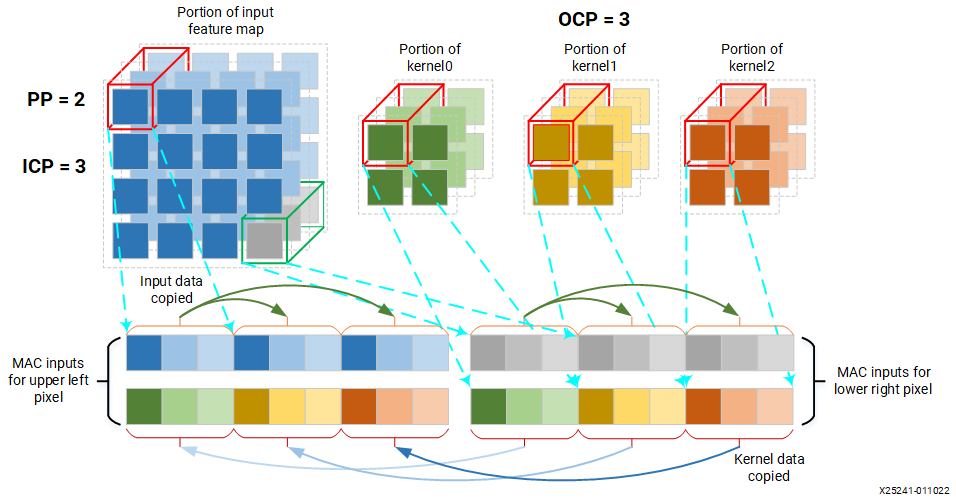# Architecture of the DPUCZDX8G - 4.1 English

## DPUCZDX8G for Zynq UltraScale+ MPSoCs Product Guide (PG338)

Document ID
PG338
Version
4.1 English

The DPUCZDX8G IP can be configured with various convolution architectures which are related to the parallelism of the convolution unit. The architectures for the DPUCZDX8G IP include B512, B800, B1024, B1152, B1600, B2304, B3136, and B4096.

There are three dimensions of parallelism in the DPUCZDX8G convolution architecture: pixel parallelism, input channel parallelism, and output channel parallelism. The input channel parallelism is always equal to the output channel parallelism (this is equivalent to channel_parallel in Table 8).

Figure 1. Visualizing the three dimensions of parallelismIn Figure 10, input channel parallelism (ICP) = 3; output channel parallelism (OCP) = 3; and pixel parallelism (PP) = 2. OCP is equivalent to the number of kernels used during a convolution computation. The pixels used in the figure are arbitrary to maintain clarity.
Note: The elements used in the computation use 1 pixel from each channel (the red cuboids in the figure). With ICP = OCP = 3 and PP = 2, the number of convolution MACs per cycle is 3 * 3 * 2 = 18.

The different architectures require different programmable logic resources. The larger architectures can achieve higher performance with more resources. The parallelism for the different architectures is listed in the following table.

Table 1. Parallelism for Different Convolution Architectures
DPUCZDX8G Architecture Pixel Parallelism (PP) Input Channel Parallelism (ICP) Output Channel Parallelism (OCP) Peak Ops (operations/per cycle)
B512 4 8 8 512
B800 4 10 10 800
B1024 8 8 8 1024
B1152 4 12 12 1152
B1600 8 10 10 1600
B2304 8 12 12 2304
B3136 8 14 14 3136
B4096 8 16 16 4096
1. In each clock cycle, the convolution array performs a multiplication and an accumulation, which are counted as two operations. Thus, the peak number of operations per cycle is equal to PP*ICP*OCP*2.